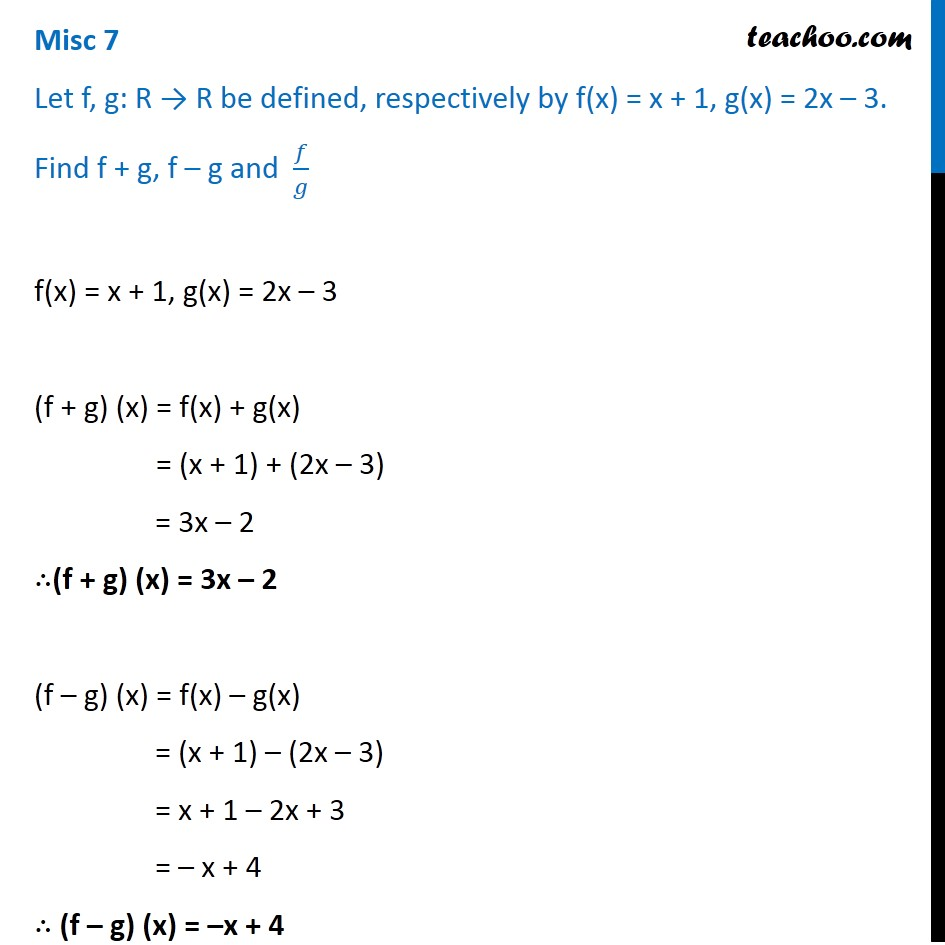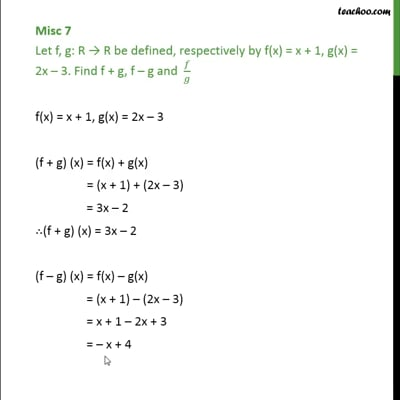Miscellaneous

Chapter 2 Class 11 Relations and Functions
Serial order wiseThis video is only available for Teachoo black users

Solve all your doubts with Teachoo Black (new monthly pack available now!)

### Transcript

Misc 7 Let f, g: R → R be defined, respectively by f(x) = x + 1, g(x) = 2x – 3. Find f + g, f – g and 𝑓/𝑔 f(x) = x + 1, g(x) = 2x – 3 (f + g) (x) = f(x) + g(x) = (x + 1) + (2x – 3) = 3x – 2 ∴(f + g) (x) = 3x – 2 (f – g) (x) = f(x) – g(x) = (x + 1) – (2x – 3) = x + 1 – 2x + 3 = – x + 4 ∴ (f – g) (x) = –x + 4